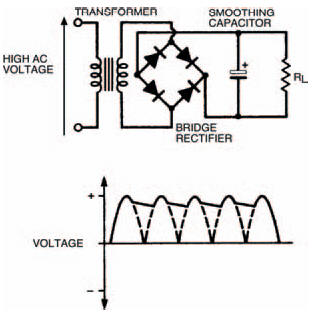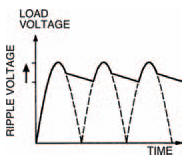# Filter tipsElectronics guide > Diodes II > Filter tips

Although we’ve managed to obtain a full-wave rectified d.c. load voltage we still have the problem that this voltage is not too steady (ideally we would like a fixed d.c. voltage which doesn’t vary at all). We can reduce the up-and-down variability of the waves by adding a capacitor to the circuit output. If you remember, a capacitor stores charges — so we can use it to average out the variation in level of the full-wave rectified d.c. voltage. Figure 7.12 shows the idea and a possible resultant waveform. This process is referred to as smoothing and a capacitor used to this effect is a smoothing capacitor. Sometimes the process is also called filtering.Figure 7.12 Levelling the rectifier d.c. with the help of a capacitor: the process is known as smoothing

You should remember that the rate at which a capacitor discharges is dependent on the value of the capacitor. So, to make sure the stored voltage doesn’t fall too far in the time between the peaks of the half cycles, the capacitor should be large enough (typically of the value of thousands of microfarads) to store enough charge to prevent this happening. Nevertheless a variation in voltage will always occur, and the extent of this variation is known as the ripple voltage, shown in Figure 7.13. Ripple voltages of the order of a volt or so are common, superimposed on the required d.c. voltage, for this type of circuit.Figure 7.13 The extent to which the d.c. is not exactly linear is known as the ripple voltage

<< Diode circuits